Select Page

# CBSE 12 Science Solutions for MCQ Maths Probability in English

CBSE 12 Science Solutions for MCQ Maths Probability in English to enable students to get Solutions in a narrative video format for the specific question.

Expert Teacher provides CBSE 12 Science Solutions for MCQ Maths Probability through Video Solutions in English language. This video solution will be useful for students to understand how to write an answer in exam in order to score more marks. This teacher uses a narrative style for a question from Probability not only to explain the proper method of answering question, but deriving right answer too.

Please find the question below and view the Solution in a narrative video format.

Question:

Solution Video in English:

You can select video Solutions from other languages also. Please check Solutions in ( Hindi )

## Similar Questions from CBSE, 12th Science, Maths, Probability

Question 1 : A class has 15 students whose ages are 14, 17, 15, 14, 21, 17, 19, 20, 16, 18, 20, 17, 16, 19 and 20 years. One student is selected in such a manner that each has the same chance of being chosen and the age X of the selected student is recorded. What is the probability of the random variable X? Find the mean.   (View Answer Video)

Question 2 : Three persons A, B and C apply for a  job of Manager in a Private company. Chance of their selection (A, B and C) are in the ratio 1:2:4. The probability that A, B, and C can introduce changes to improve profits of company are 0.8, 0.5 and 0.3 respectively, if the changes does not take place, find the probability that is due to the appointment of C.    (View Answer Video)

Question 3 : A pair of dice is thrown 4 times. If getting a doublet is considered a success, find the probability distribution of number of successes. Hence, find the mean of the distribution.  (View Answer Video)

Question 4 : If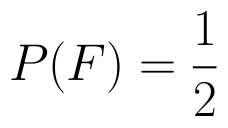and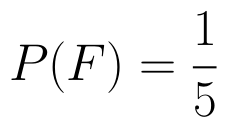find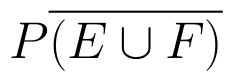if E and F are independent events.  (View Answer Video)

Question 5 : If A and B are two events such that P(A)=0.3, P(B)=0.6 and P(B/A)=0.5, find P(A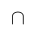B). (View Answer Video)

### Linear Programming

Question 1 : The objective function is maximum or minimum, which lies on the boundary of the feasible region. (View Answer Video)

### Differential Equations

Question 1 : If x cos(a + y) = cos y, then prove that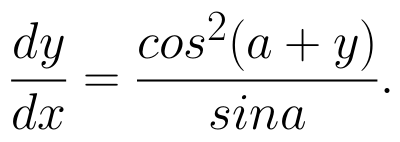Hence show that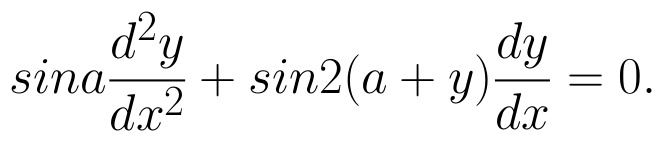(View Answer Video)

Question 2 : Write the differential equation representing the curve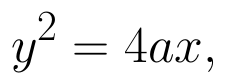where a is an arbitrary constant. (View Answer Video)

Question 3 : Determine degree of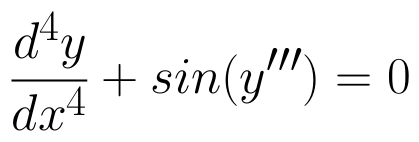(View Answer Video)

Question 4 : Write the degree of the differential equation :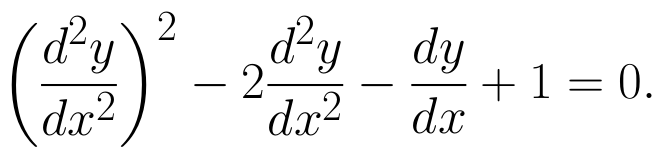(View Answer Video)

Question 5 : Find the particular solution of the differential equation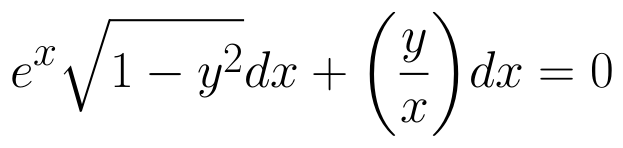given that y = 1 when x = 0. (View Answer Video)

### Matrices

Question 1 : Find the value of X, if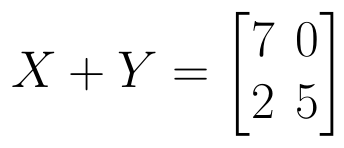and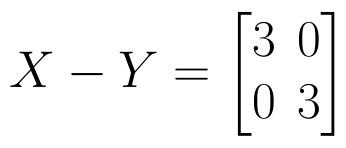. (View Answer Video)

Question 2 :  Find the value of t, if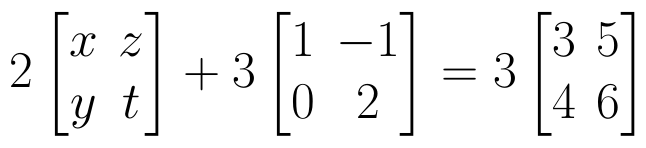(View Answer Video)

Question 3 : Compute: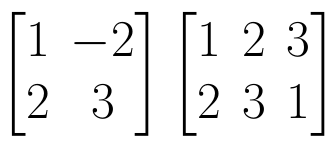. (View Answer Video)

Question 4 : If,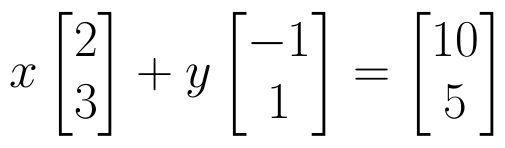find the value of x. (View Answer Video)

Question 5 : Find the value of x,  from the equation: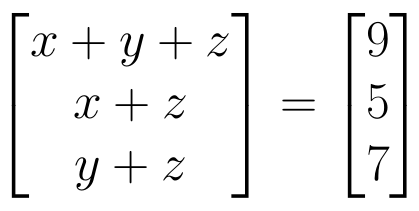. (View Answer Video)